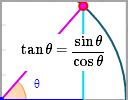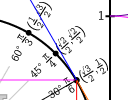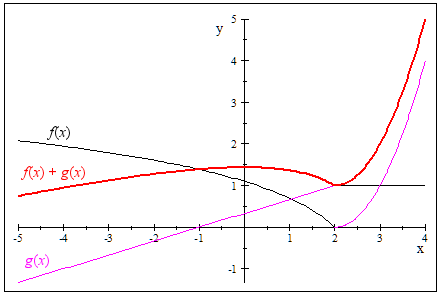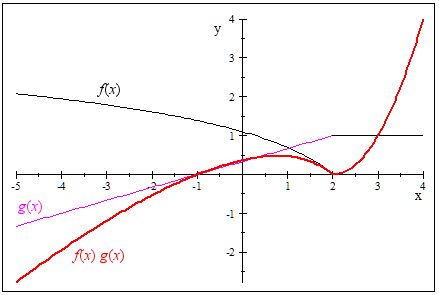Search IntMath
Close

450+ Math Lessons written by Math Professors and Teachers

5 Million+ Students Helped Each Year

1200+ Articles Written by Math Educators and Enthusiasts

Simplifying and Teaching Math for Over 23 Years

# IntMath Newsletter: Pollution, trig ratios and infinity

By Murray Bourne, 04 Jul 2013

4 Jul 2013

1. Math of air pollution
2. Trig ratios: updated interactive
3. Resource: Touch Mathematics
4. Math puzzles
5. Friday math movie: How big is infinity?
6. Final thought: Imagination

## 1. Math of air pollutionHow do we compare pollution caused by different substances and give meaningful health advisories? Singapore's current haze season gives an example. Math of air pollution

## 2. Trig ratios: updated interactiveHere's an interactive graph where you can investigate some important trigonometric identities. Trig ratios: updated interactive

## 3. Resource: Touch MathematicsTouch Mathematics is an interesting new site containing 2 interactive graphs - one for trigonometry (relating the unit circle with the graphs of trigonometric functions) and derivatives (giving the graphs of the first and second derivatives of some common functions).

There's a lot going on in each graph (probably too much), but it's possible to turn on and off the graphs so you can see better. (In the trigonometry one, click on the function names under the circle to toggle the graphs.)

## 4. Math puzzles

The puzzle in the last IntMath Newsletter involved finding the limit of the sum and product of 2 given functions.

The interesting thing about this question is that we normally need to assume the limits exist for the given functions before we can say the limit of the sum is the sum of the limits, and likewise for the products. (See a typical explanation here: Limit Properties.)

In the question, the limit at x = 2 does not exist for either of the functions.

But it appears the limits of the sum and product do exist. The limit of the sum is 1 as we approach from either the left or right, and for the product the limit is 0 as we approach from the left or right, as you can see in the graphs below:The functions (in black and magenta) and their sum, shown in red.

And now for the product:The functions (in black and magenta) and their product, shown in red.

Your responses: Some of you said because the limits don't exist, then the limits of the sums and products don't exist (Nosipho, Sachin).

Others of you (Guido, Douglas, hamid and Tim) agreed with the values shown in the graphs above.

So this is an interesting case. Even though the limits of the component functions don't exist at x = 2, the limits of the sum and product do exist.

New Puzzle

We are given an integer x and told it is the square of a square, and that it has 18 as a factor. What is the least value of x?

## 5. Friday math movie: How big is infinity?This is a really interesting mind experiment. You may even find yourself re-thinking your concept of infinity. Friday math movie: How big is infinity?

## 6. Final thought: Imagination

Sadly, "imagination" is not a word we often hear used in math classrooms. All too often the main thrust is making sure students get the one correct answer. I think math would be a lot more interesting if imagination had more prominence.

This leads me to the following quote by Albert Einstein. If he hadn't used imagination, he would never have come up with the Theory of Relativity.

Your imagination is a preview to life's coming attractions. [Albert Einstein]

Until next time, enjoy whatever you learn.

1. Deborah Tayler says:

1296 is my answer, but I'm sure I'm missing some clever trick to find something smaller!

2. INFINITY says:

x has a factor of (2^1)^(3^2).
Square of square is power of four.
To make x square of a square, power of each prime factor must be power of four.
x should have a factor of (2^4)*(3^4)= 6^4= 1296
x = 1296k where k is power of four of a positive integer
Least value of x has least value of k. So, k= 1^4= 1,
x=1296

3. Nqoba says:

Answer to the teaser is 1296 that is 6^4

4. Alan Johnstone says:

x=36
x=18=3*3*2
the smallest additional factor that will make this a perfect square is 2
3*3*2*2=36

5. devanie says:

6*6 = 36
36*36= 1296
1296/18 = 72
Therefore x = 1296
Also, 36 is a square number that has a factor of 18. Hence 36*36 =1296

6. Francis Kisner says:

Since x = n^4 and x = 18y = 3*3*2*y, the smallest integer value for x must be 2^4 * 3^4

7. Shantosh says:

The answer to the puzzle is 1296.

dear sir,
the answer I got is 1296 which is 4th power of 6
reason: 18=3*3*2 we need every prime to repeat 4 times for it to be
4th power of some number. so 3*3*2*3*3*2*2*2=1296=6^4

### Comment Preview

HTML: You can use simple tags like <b>, <a href="...">, etc.

To enter math, you can can either:

1. Use simple calculator-like input in the following format (surround your math in backticks, or qq on tablet or phone):
a^2 = sqrt(b^2 + c^2)
(See more on ASCIIMath syntax); or
2. Use simple LaTeX in the following format. Surround your math with $$ and $$.
$$\int g dx = \sqrt{\frac{a}{b}}$$
(This is standard simple LaTeX.)

NOTE: You can mix both types of math entry in your comment.